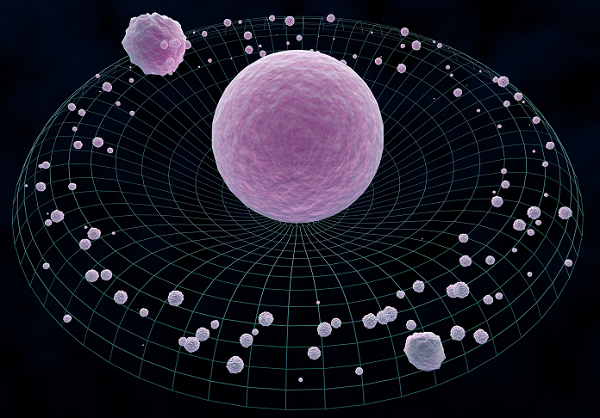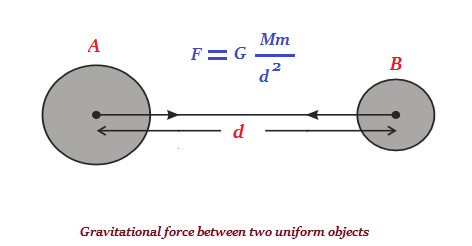# Physics - Gravitation

## Introduction

• All celestial bodies those found in the universe attract each other and the force of attraction among these bodies is called as the gravitational force.## Universal Law of Gravitation

• Every object in the universe has the property to attract every other object with a force which is directly proportional to the product of their masses and inversely proportional to the square of the distance between them (see the image given below).• F = force of attraction between two the objects ‘A’ & ‘B’

• M = mass of ‘A’

• m = mass of ‘B’

• d2 = the square of the distance between ‘A’ & ‘B’

• G = is the constant of proportionality and is known as the universal gravitation constant.

• The SI unit of G is N m2 kg–2. It is obtained by substituting the units of force, distance and mass (as given in the following equation −

$$G = \frac{Fd^2}{M \times m}$$

• Henry Cavendish had calculated the value of ‘G’ as 6.673 × 10–11 N m2 kg–2.

• Henry Cavendish had used a sensitive balance to find the value of ‘G.’

## Significance of Universal Law of Gravitation

• Following are the salient significance of the Universal Law of Gravitation −

• It explains the force that binds all objects (including human beings) to the earth

• It describes the motion of the moon around the earth

• It explains the motion of planets around the Sun

• It clarifies the tides due to the moon and the Sun

## Free Fall

• Whenever an object falls towards the earth, it involves an acceleration; this acceleration is produces due to the earth’s gravitational force.• The acceleration, produces due to the earth’s gravitational force, is known as the acceleration due to the gravitational force of the earth (or acceleration due to gravity).

• The acceleration produces due to the gravitational force is denoted by g.

• As the radius of the earth increases towards the equator (from the poles) the value of ‘g’ becomes greater at the poles than at the equator.

## The Value of g

• Value of g is calculated as −

$$g = G\frac{M}{R^2}$$

• G = universal gravitational constant, which is = 6.7 × 10–11 N m2 kg-2

• M = mass of the earth, which is = 6 × 1024 kg

• R = radius of the earth, which is = 6.4 × 106 m

• So,

$$g = \frac{6.7 \: \times 10^{-11} \: Nm^2 \: kg^{-2} \: \times \: 6 \: \times 10^{24} \: kg}{(6.4 \: \times 10^6 \: m)^2}$$

$=9.8 \: m \: s^{-2}$

• So, the value of acceleration due to gravity of the earth (g) is 9.8 m s-2.